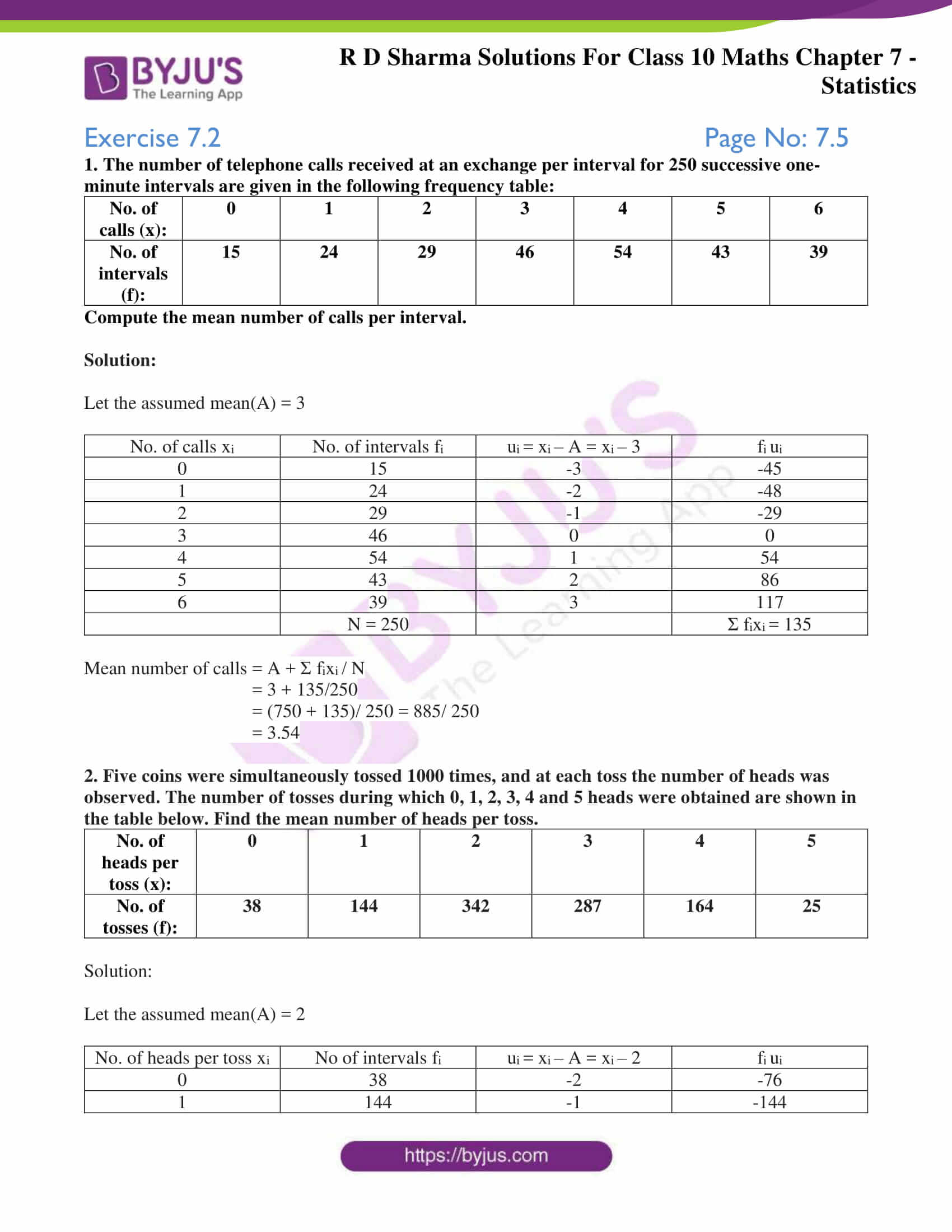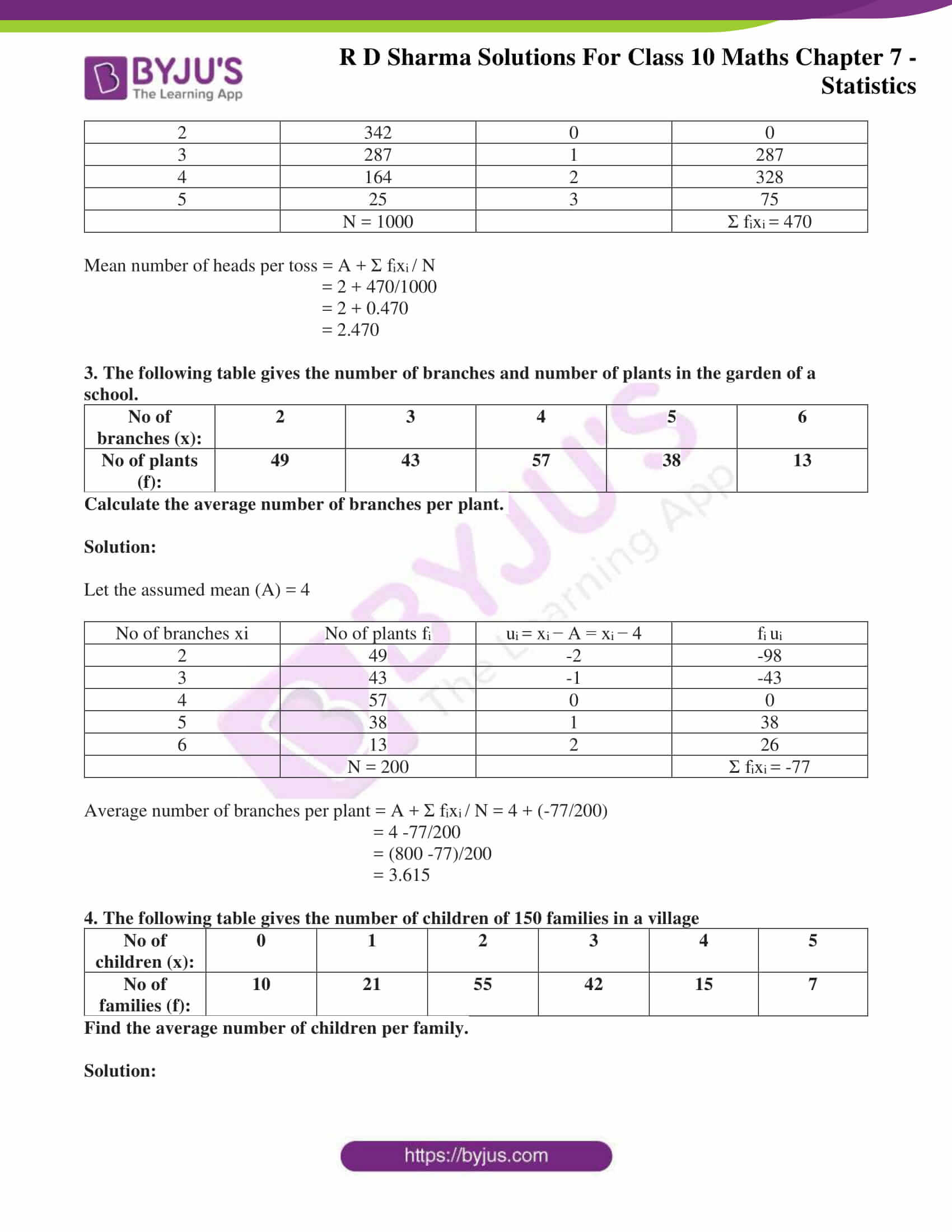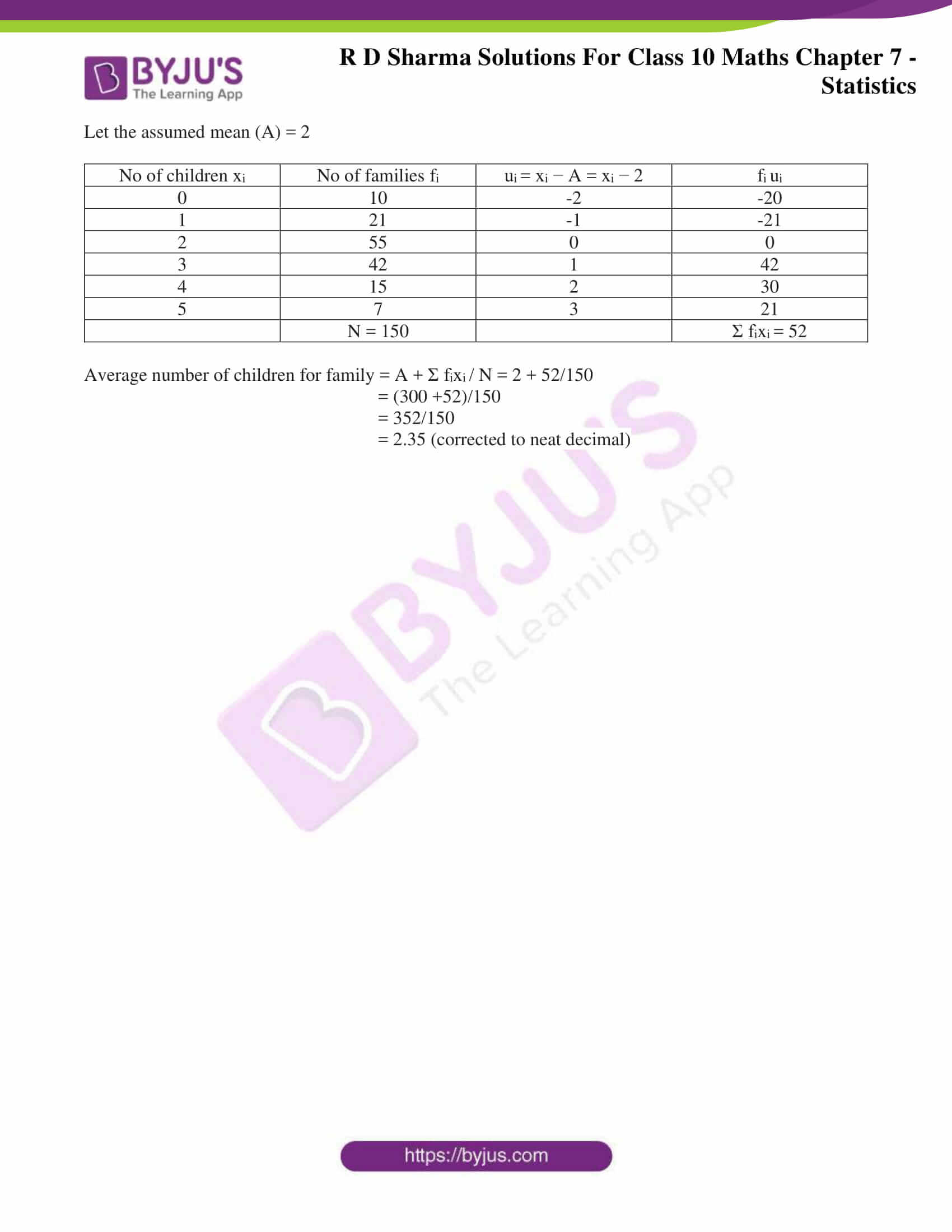# RD Sharma Solutions for Class 10 Maths Chapter 7 Statistics Exercise 7.2

In order to overcome minor errors in finding AM, the step deviation method is considered more appropriate for calculating the AM. Students wanting to build confidence in solving Class 10 Mathematics can access the RD Sharma Solutions Class 10. For any doubt clearing and explanations regarding this exercise, download RD Sharma Solutions for Class 10 Maths Chapter 7 Statistics Exercise 7.2, PDF provided below.

## RD Sharma Solutions for Class 10 Maths Chapter 7 Statistics Exercise 7.2 Download PDF### Access RD Sharma Solutions for Class 10 Maths Chapter 7 Statistics Exercise 7.2

1. The number of telephone calls received at an exchange per interval for 250 successive one- minute intervals are given in the following frequency table:

 No. of calls (x): 0 1 2 3 4 5 6 No. of intervals (f): 15 24 29 46 54 43 39

Compute the mean number of calls per interval.

Solution:

Let the assumed mean(A) = 3

 No. of calls xi No. of intervals fi ui = xi – A = xi – 3 fi ui 0 15 -3 -45 1 24 -2 -48 2 29 -1 -29 3 46 0 0 4 54 1 54 5 43 2 86 6 39 3 117 N = 250 Σ fixi = 135

Mean number of calls = A + Σ fixi / N

= 3 + 135/250

= (750 + 135)/ 250 = 885/ 250

= 3.54

2. Five coins were simultaneously tossed 1000 times, and at each toss the number of heads was observed. The number of tosses during which 0, 1, 2, 3, 4 and 5 heads were obtained are shown in the table below. Find the mean number of heads per toss.

 No. of heads per toss (x): 0 1 2 3 4 5 No. of tosses (f): 38 144 342 287 164 25

Solution:

Let the assumed mean(A) = 2

 No. of heads per toss xi No of intervals fi ui = xi – A = xi – 2 fi ui 0 38 -2 -76 1 144 -1 -144 2 342 0 0 3 287 1 287 4 164 2 328 5 25 3 75 N = 1000 Σ fixi = 470

Mean number of heads per toss = A + Σ fixi / N

= 2 + 470/1000

= 2 + 0.470

= 2.470

3. The following table gives the number of branches and number of plants in the garden of a school.

 No of branches (x): 2 3 4 5 6 No of plants (f): 49 43 57 38 13

Calculate the average number of branches per plant.

Solution:

Let the assumed mean (A) = 4

 No of branches xi No of plants fi ui = xi − A = xi − 4 fi ui 2 49 -2 -98 3 43 -1 -43 4 57 0 0 5 38 1 38 6 13 2 26 N = 200 Σ fixi = -77

Average number of branches per plant = A + Σ fixi / N = 4 + (-77/200)

= 4 -77/200

= (800 -77)/200

= 3.615

4. The following table gives the number of children of 150 families in a village

 No of children (x): 0 1 2 3 4 5 No of families (f): 10 21 55 42 15 7

Find the average number of children per family.

Solution:

Let the assumed mean (A) = 2

 No of children xi No of families fi ui = xi − A = xi − 2 fi ui 0 10 -2 -20 1 21 -1 -21 2 55 0 0 3 42 1 42 4 15 2 30 5 7 3 21 N = 150 Σ fixi = 52

Average number of children for family = A + Σ fixi / N = 2 + 52/150

= (300 +52)/150

= 352/150

= 2.35 (corrected to neat decimal)